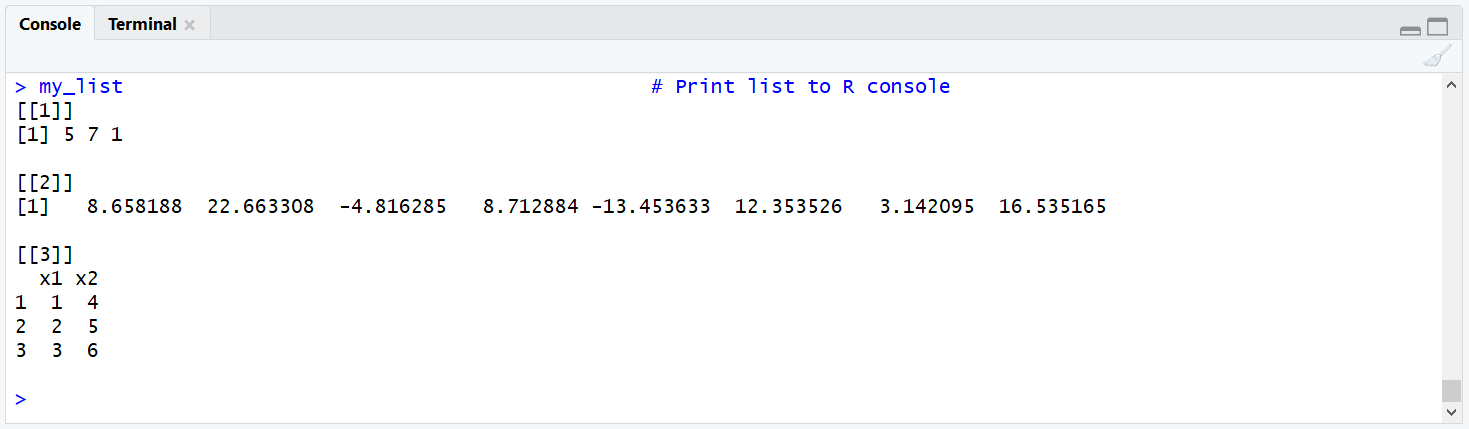# The length Function in R (3 Examples for Vector, List & String)

Basic R Syntax:

`length(x)`

The length function returns the length of R objects such as vectors, lists, or strings (find a little trick in Example 3). The R code above illustrates how to apply length in R.

In this article, I’m going to provide 3 examples for the application of the length command in R. So without further ado, let’s get started…

## Example 1: Check Length of Vector in R

Before we can start, we need to create a vector or array in R:

`x <- c(8, 17, 23, 93, - 20, 15, 13, 55, 29, - 84)  # Example vector in R`

Now, we can apply the length R command to this vector:

```length(x)                                          # Apply length function
# 10```

The length function returns the value 10 to the RStudio console – The length of our vector is 10.

By the way: I have also published a video on my YouTube channel, which is explaining Example 1. You can check it out here:

## Example 2: Find Length of List

As in the example before, we have to create some example data first:

```set.seed(1357)                                     # Set seed for reproducibility
my_list <- list()                                  # Create empty list
my_list[] <- c(5, 7, 1)                         # List element 1
my_list[] <- rnorm(8, 5, 10)                    # List element 2
my_list[] <- data.frame(x1 = 1:3, x2 = 4:6)     # List element 3
my_list                                            # Print list to R console```Graphic 1: Output of the Example List in the RStudio Console.

Our list consists of three entries: two numeric vectors at position 1 and 2 as well as of a data frame at position 3.

For such list objects, you can apply the length R function in the same manner as before:

```length(my_list)                                    # Get length of list
# 3```

Note: The length function returns the number of entries of our list, not the length of each element of the list. If you want to get the length of a single list element, you can use the following R code:

```length(my_list[])                               # Get length of second list entry
# 8```

The length of the second list element is 8.

## Example 3: Get Length of String (Little Trick Needed)

Even though you have to use a little trick, length can also be applied to a string (i.e. a data object of the class character). Consider the following example string:

`x_string <- "Hello this is a string"               # Create example string`

If you apply length as before, you will probably not get the result you want:

```length(x_string)                                   # Basic application of length()
# 1```

R returns 1 – the length of this data object and not the number of characters within the string.

If you want to count the number of characters within a string, you have to use length in combination with the unlist and strsplit functions:

```length(unlist(strsplit(x_string, "")))             # Combine length, unlist & strsplit
# 22```

Our string consists of 22 character values.

That said, there is a less complicated way to get the number of character values within a string. You can simply use the nchar function:

```nchar(x_string)                                    # Apply nchar function in R
# 22```

Much easier, but the same result!

## The length Function in Action: Video Examples for length() and Related Commands

For more examples of the length() function in R, have a look at the following video of the DataCamp YouTube channel.